Anna Grossnickle Hines                                                                                 Home    Guide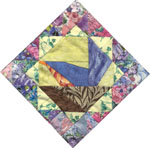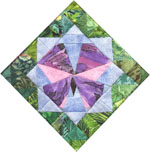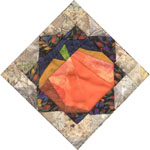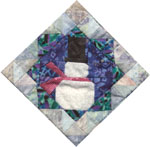Quilts in the Classroom Let's Do Math to PIECES! using the book, PIECES: A YEAR IN POEMS AND QUILTS The amazing shrinking squares! Can you do area? Look at the difference between how much fabric is in each quilt and how much fabric ends up showing.  Isn’t that amazing?  My editor said she didn’t believe it even when I showed her the pictures.  Want to figure it out?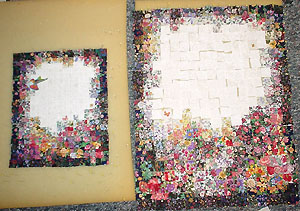Area is the measurement of the surface of something. Usually we measure area in square inches, squares centimeters, square feet, square meters, square yards, square miles or square kilometers. My fabric squares are so small well have to use a smaller measurement.  Lets use square eighths, tiny little squares 1/8 of an inch on each side. Each one of the squares I cut out for my quilts is 11/8 inches on each side.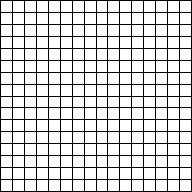Draw a square on this grid that is 11/8 inches on each side.  Print grids HTML or PDF. The lines on the grid are 1/8 inch apart. The square you draw will be the same size as one of my fabric squares. What is the area of the square? Another way to ask this is, how many little square 1/8 inches are in the 11/8 inch square? How can you find out? You could count them the little square eighths. …or you could multiply the length times the width.  That is the formula for finding the area of a rectangle. [ A = L x W] Which way is faster? Can you find the are using the formula, A = L x W ? Hint: How many eighths are on each side? The length is ___ eighths and the width is ___ eighths. So the area is___square eighths. When I sew a square into the quilt each seam is ¼ inch wide.  This means that ¼ inch gets taken off of each side of each square. Can you show this on the grid? Hint: Draw a line ¼ inch inside each edge of the square. How many eighths are in ¼? The square inside is all that will be left showing in the quilt. How long is it on each side? How many square eighths is it? How many square eighths are NOT showing? Do more than half show or less than half? What fraction of the area of the square ends up showing? Can you figure out the percentage that is showing? Hint: Divide the number of square eighths that show by the number of square eighths in the square before it’s sewn. Are you amazed?  Maybe I should show this math to my editor! Do More Math to Pieces... Guess How Many?   Fun with geometric shapes and numbers Half-square Triangle Designs: Glossary of Quilting A Bit of History Quilts in the Classroom Quilts, Dolls and Books Quilter's Page Pieces Top of Page Guide Home